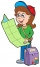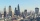# Tourist

A tourist walked an average speed of 3.5 km/h route in 6 hours. Calculate how many hours he would have passed at an average speed of 5.5 km/h.

Correct result:

t =  3.818 h

#### Solution:

$v_{1}=3.5 \ \text{km/h} \ \\ t_{1}=6 \ \text{h} \ \\ v_{2}=5.5 \ \text{km/h} \ \\ \ \\ s=v_{1} \cdot \ t_{1}=3.5 \cdot \ 6=21 \ \text{km} \ \\ \ \\ t=s/v_{2}=21/5.5=\dfrac{ 42 }{ 11 }=3.818 \ \text{h}$Our examples were largely sent or created by pupils and students themselves. Therefore, we would be pleased if you could send us any errors you found, spelling mistakes, or rephasing the example. Thank you!

Please write to us with your comment on the math problem or ask something. Thank you for helping each other - students, teachers, parents, and problem authors.Tips to related online calculators
Check out our ratio calculator.
Do you want to convert length units?
Do you want to convert velocity (speed) units?
Do you want to convert time units like minutes to seconds?
Most natural application of trigonometry and trigonometric functions is a calculation of the triangles. Common and less common calculations of different types of triangles offers our triangle calculator. Word trigonometry comes from Greek and literally means triangle calculation.

## Next similar math problems:

• The touristThe tourist walked a quarter of the way on the first day, a third of the rest on the second day, and 20 km on the last day. How many km did he walk in three days?
• Tourist routeHow long is the tourist route when tourists crossed four-sevenths of the way on foot, crossed the bus twice less than on foot and passed the last 14 kilometers by boat.
• Cyclist 12What is the average speed of a cycle traveling at 20 km in 60 minutes in km/h?
• Average heightThere are twice as many girls in the class as there are boys. The average height of girls is 177 cm, boys 186 cm. What is the average height of students in this class?
• FighterA military fighter flies at an altitude of 10 km. From the ground position, it was aimed at an altitude angle of 23° and 12 seconds later at an altitude angle of 27°. Calculate the speed of the fighter in km/h.Calculate the surface of a quadrilateral prism according to the input: Area of the diamond base S1 = 2.8 m2, length of the base edge a = 14 dm, height of the prism 1,500 mm.
• Circle and squareAn ABCD square with a side length of 100 mm is given. Calculate the radius of the circle that passes through the vertices B, C and the center of the side AD.
• Wire fenceThe wire fence around the garden is 160 m long. One side of the garden is three times longer than the other. How many meters do the individual sides of the garden measure?
• Lookout towerHow high is the lookout tower? If each step was 3 cm lower, there would be 60 more of them on the lookout tower. If it was 3 cm higher again, it would be 40 less than it is now.
• The planThe plan of the housing estate is in three scales 1: 5000,1: 10000,1: 15000. The distance between two points on a plan with a scale of 1: 10000 is 12 cm. What is this distance on the other two plans? What is this distance?
• Divide in ratioLine segment AB 12 cm long divide in a ratio of 5: 3. How long are the individual parts?
• SailingSolve the following problem graphically. The fishing boat left the harbor early in the morning and set out to the north. After 12 km of sailing, she changed course and continued 9 km west. Then she docked and launched the nets. How far was she from the pl
• Cutting coneA cone with a base radius of 10 cm and a height of 12 cm is given. At what height above the base should we divide it by a section parallel to the base so that the volumes of the two resulting bodies are the same? Express the result in cm.
• Observation towerFrom the observation tower at a height of 105 m above sea level, the ship is aimed at a depth angle of 1° 49´. How far is the ship from the base of the tower?
• Length of the arcWhat is the length of the arc of a circle k (S, r=68mm), which belongs to a central angle of 78°?
• My fatherMy father cut 78 slats on the fence. The shortest of them was 97 cm long, the longer one was 102 cm long. What was the total length of the slats in cm?
• Identical cubesFrom the smallest number of identical cubes whose edge length is expressed by a natural number, can we build a block with dimensions 12dm x 16dm x 20dm?